# Genus two surface

Jump to: navigation, search
This article is about a particular topological space (uniquely determined up to homeomorphism)|View a complete list of particular topological spaces

## Definition

This topological space, denoted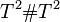$T^2 \# T^2$ or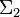$\Sigma_2$, is defined in the following equivalent ways:

1. It is the connected sum of two copies of the 2-torus.
2. It is the compact orientable surface of genus$2$.

## Topological space properties

Property Satisfied? Is the property a homotopy-invariant property of topological spaces? Explanation Corollary properties satisfied/dissatisfied
manifold Yes No By definition as a connected sum of manifolds satisfies: metrizable space, second-countable space, and all the separation axioms down from perfectly normal space and monotonically normal space, including normal, completely regular, regular, Hausdorff, etc.
path-connected space Yes Yes By definition as a connected sum of connected manifolds. satisfies: connected space, connected manifold, homogeneous space (via connected manifold, see connected manifold implies homogeneous)
simply connected space No Yes Fundamental group is nontrivial, see homotopy of compact orientable surfaces dissatisfies: simply connected manifold
rationally acyclic space No Yes The second homology group is isomorphic to the group of integers, hence it is nontrivial and has nontrivial torsion-free part. See homology of spheres dissatisfies: acyclic space, weakly contractible space, contractible space
space with Euler characteristic zero No Yes The Euler characteristic is -2, see homology of compact orientable surfaces
space with Euler characteristic one No Yes The Euler characteristic is -2, see homology of compact orientable surfaces
compact space Yes No connected sum of compact manifolds satisfies: compact manifold, compact polyhedron, polyhedron (via compact manifold), compact Hausdorff space, and all properties weaker than compactness

## Algebraic topology

### Homology

Further information: homology of compact orientable surfaces

The homology groups over the integers are as follows:$H_k(\Sigma_2;\mathbb{Z}) = \lbrace\begin{array}{rl} \mathbb{Z}, & \qquad k = 0,2 \\ \mathbb{Z}^4, & \qquad k = 1 \\ 0, & \qquad k > 2 \\\end{array}$

More generally, with coefficients in a module$M$, the homology groups are:$H_k(\Sigma_2;\mathbb{Z}) = \lbrace\begin{array}{rl} M, & \qquad k = 0,2 \\ M^4, & \qquad k = 1 \\ 0, & \qquad k > 2 \\\end{array}$

The reduced homology looks the same except that the zeroth homology groups/modules are now zero.

### Cohomology

Further information: cohomology of compact orientable surfaces

The cohomology groups over the integers are as follows: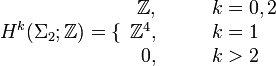$H^k(\Sigma_2;\mathbb{Z}) = \lbrace\begin{array}{rl} \mathbb{Z}, & \qquad k = 0,2 \\ \mathbb{Z}^4, & \qquad k = 1 \\ 0, & \qquad k > 2 \\\end{array}$

More generally, with coefficients in a module$M$, the cohomology groups are:$H^k(\Sigma_2;\mathbb{Z}) = \lbrace\begin{array}{rl} M, & \qquad k = 0,2 \\ M^4, & \qquad k = 1 \\ 0, & \qquad k > 2 \\\end{array}$

### Homology-based invariants

Invariant General description Description of value for genus$g$ surface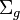$\Sigma_g$ Description of value for genus two surface$\Sigma_2$
Betti numbers The$k^{th}$ Betti number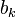$b_k$ is the rank of the torsion-free part of the$k^{th}$ homology group.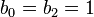$b_0 = b_2 = 1$,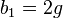$b_1 = 2g$, all higher$b_k$ are zero$b_0 = b_2 = 1$,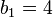$b_1 = 4$
Poincare polynomial Generating polynomial for Betti numbers$1 + 2gx + x^2$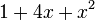$1 + 4x + x^2$
Euler characteristic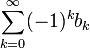$\sum_{k=0}^\infty (-1)^k b_k$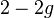$2 - 2g$ -2

### Homotopy groups

Further information: homotopy of compact orientable surfaces